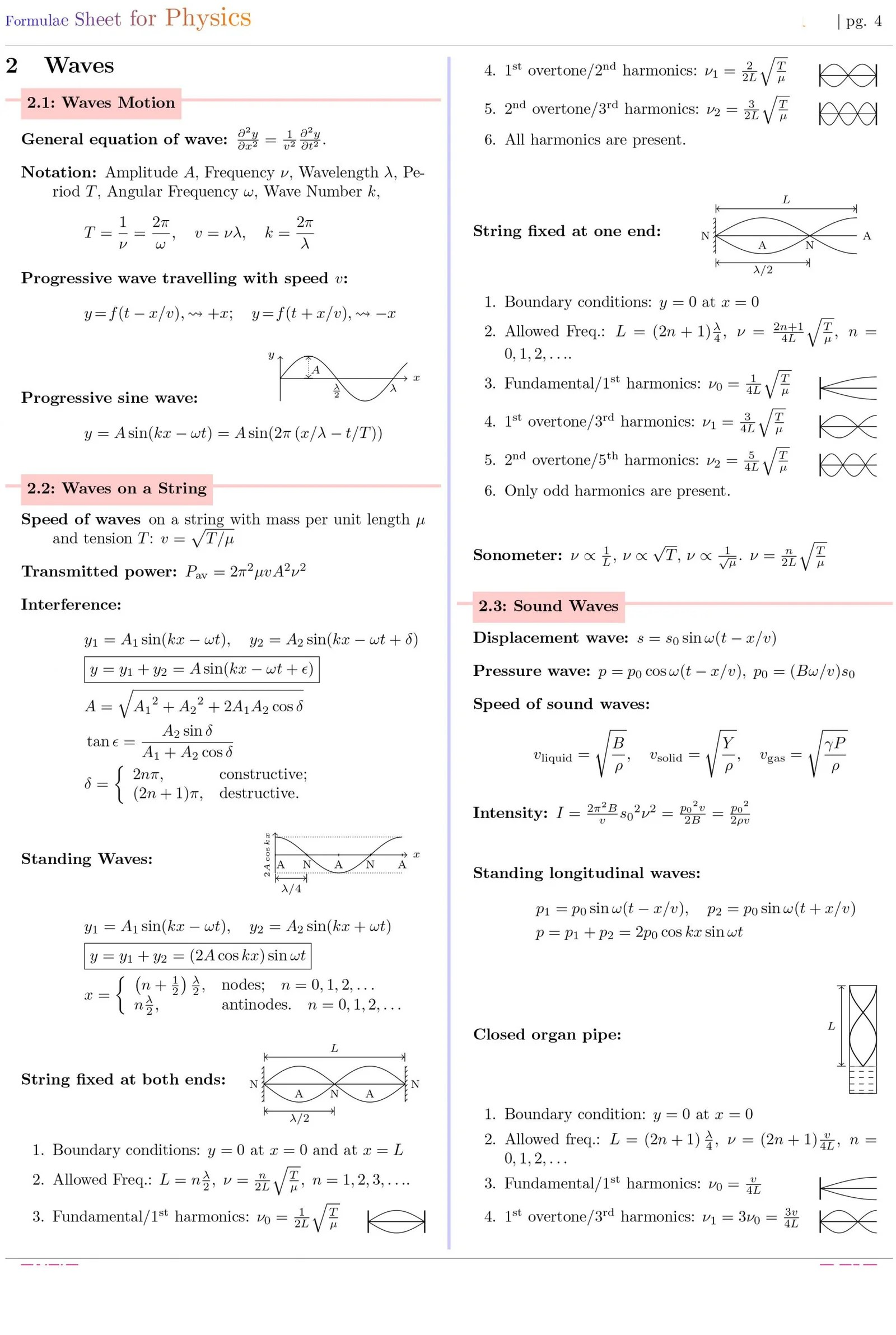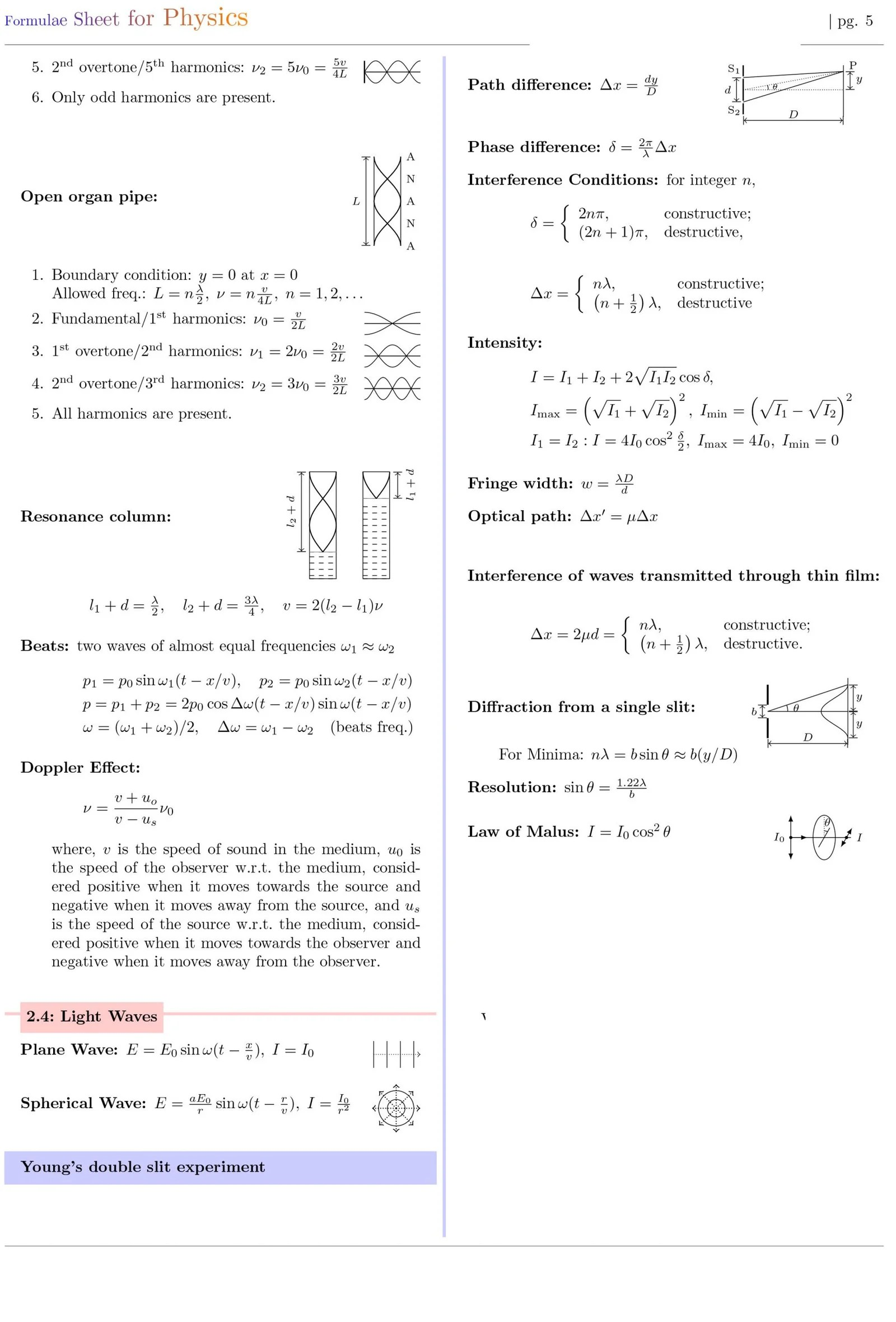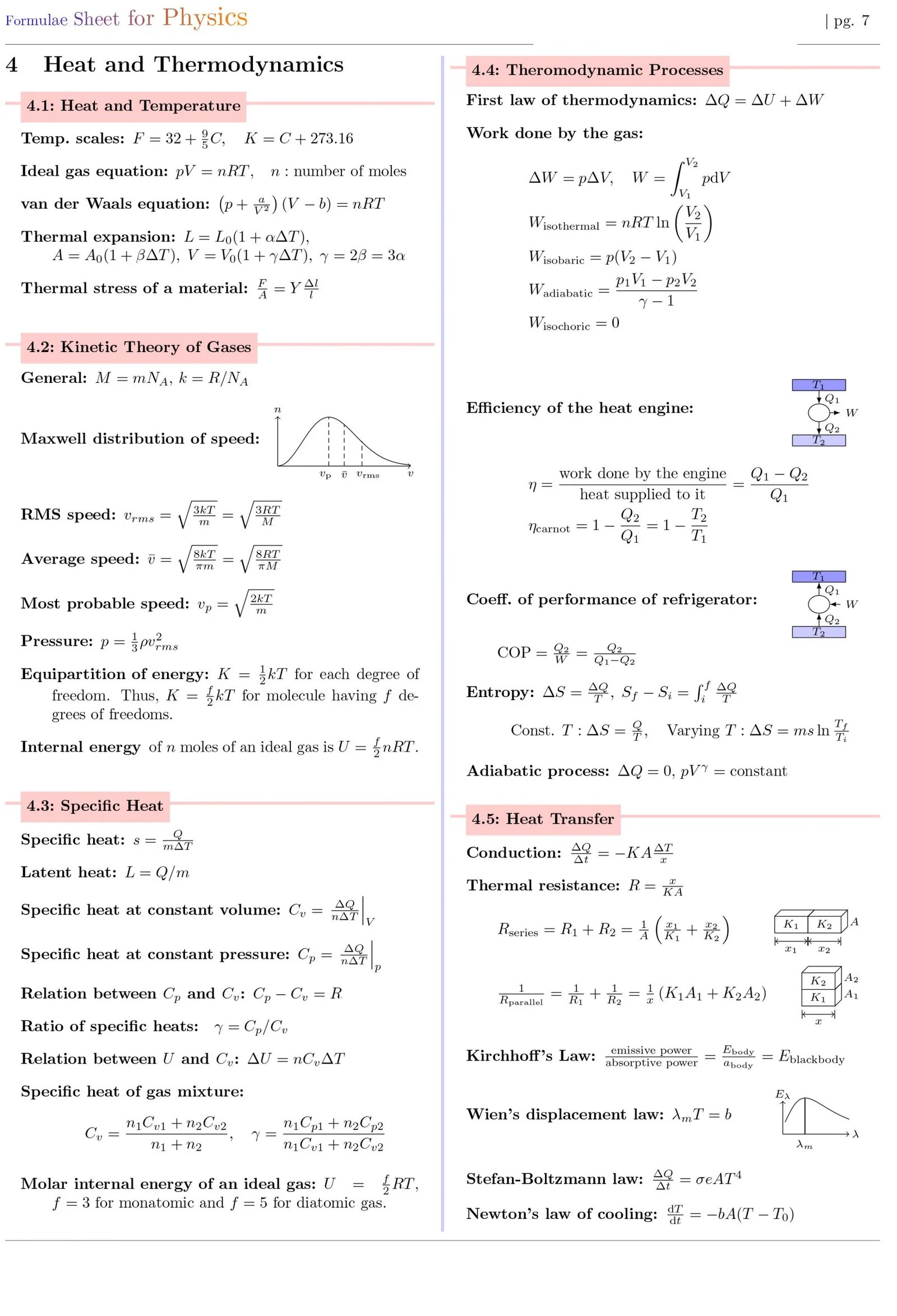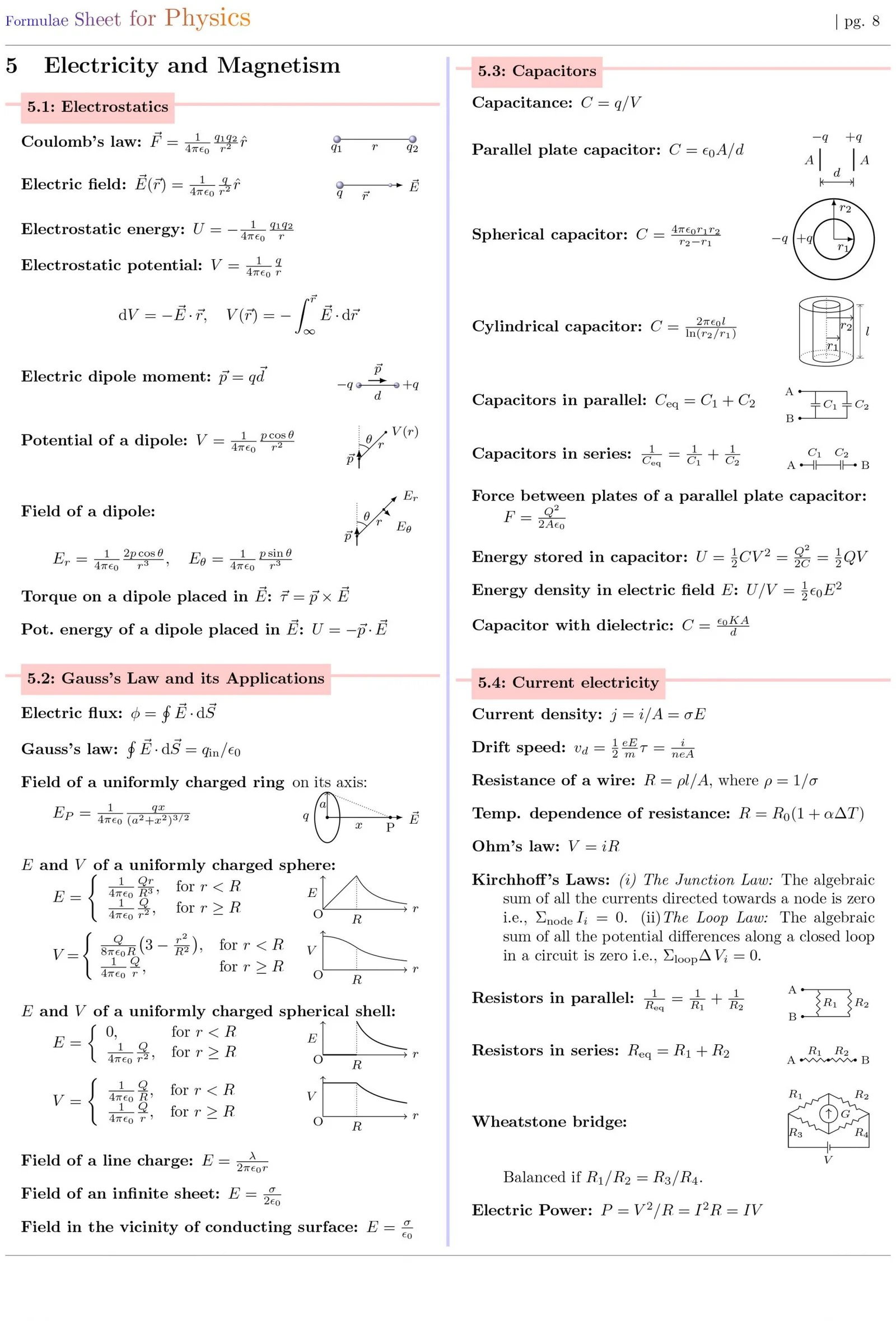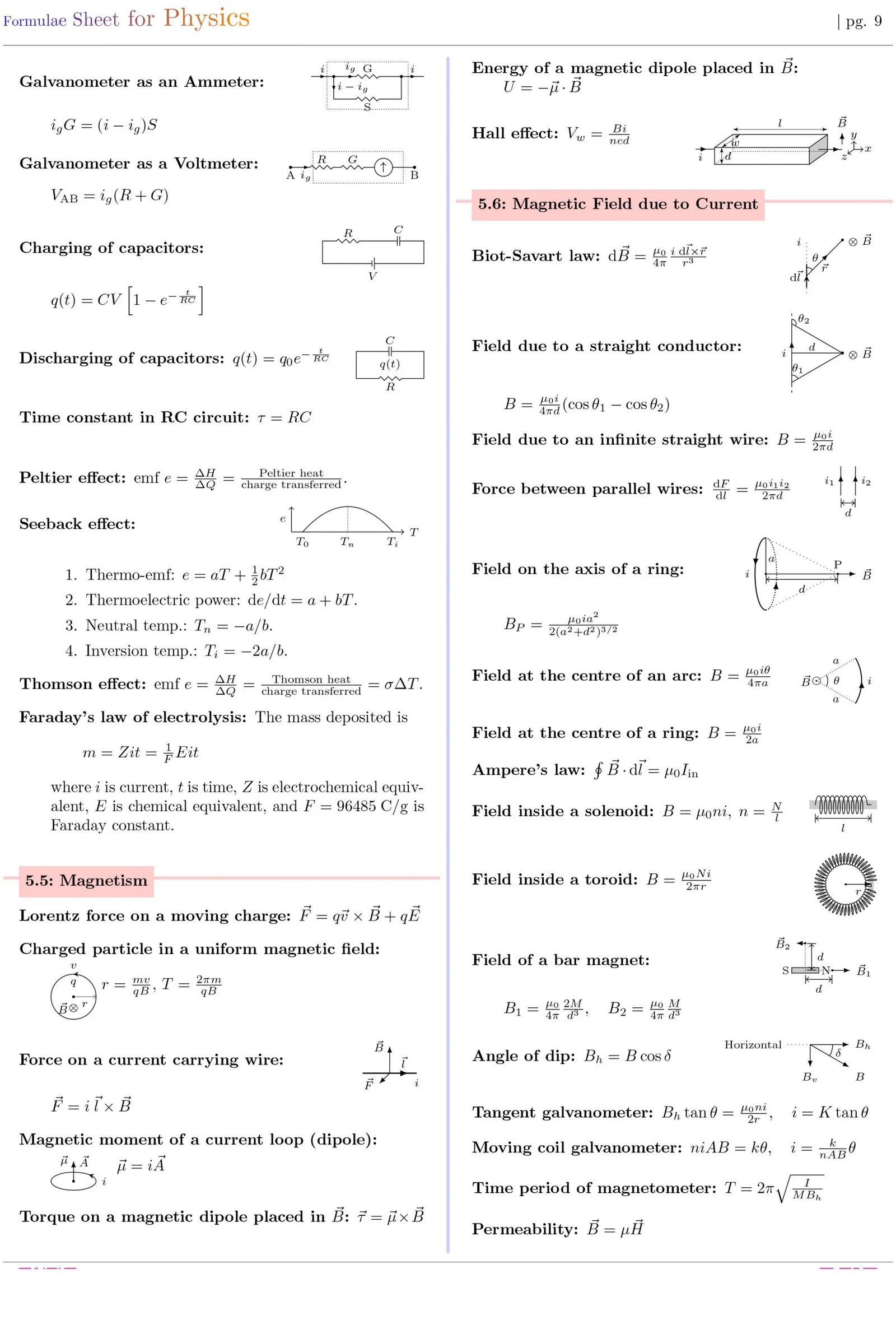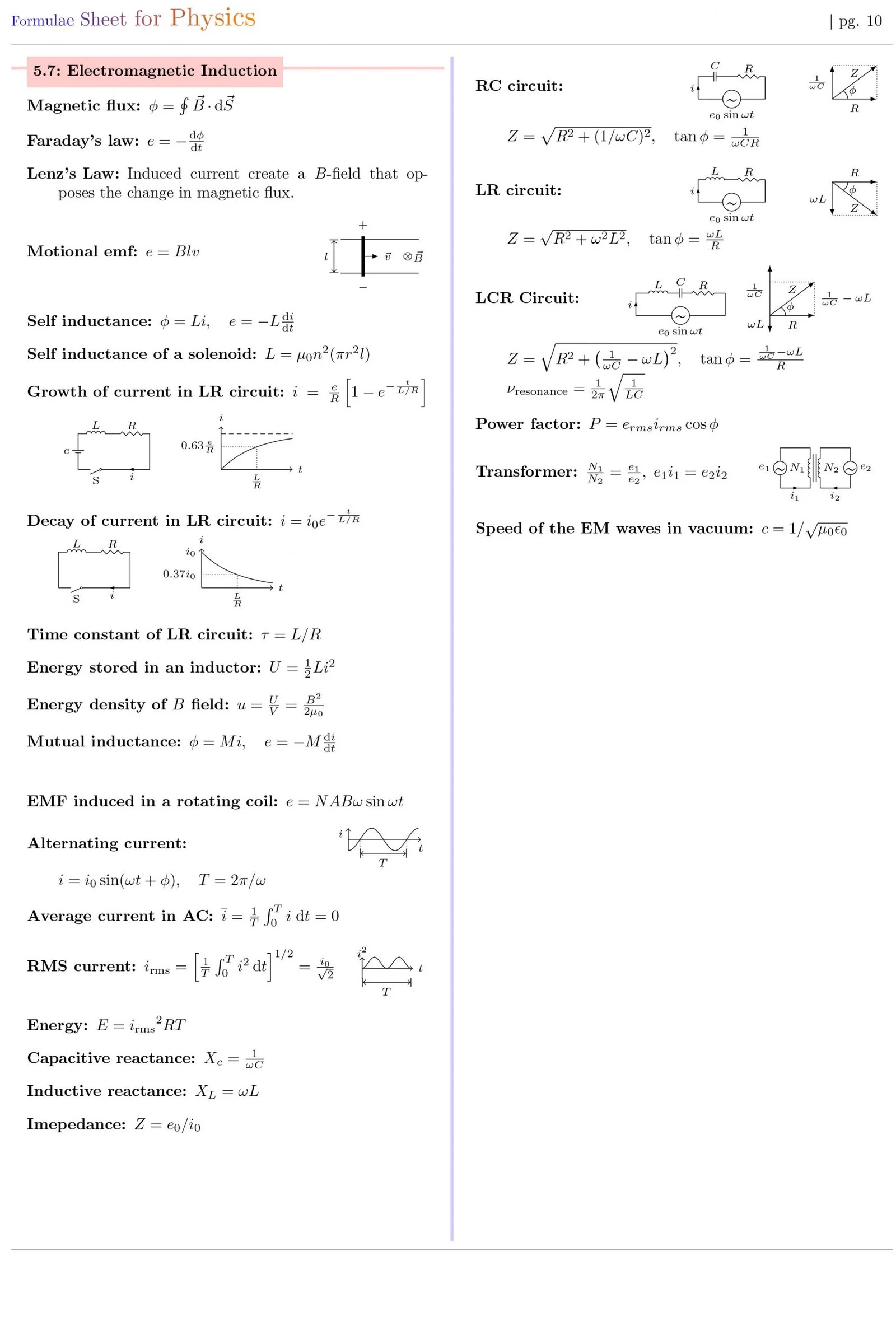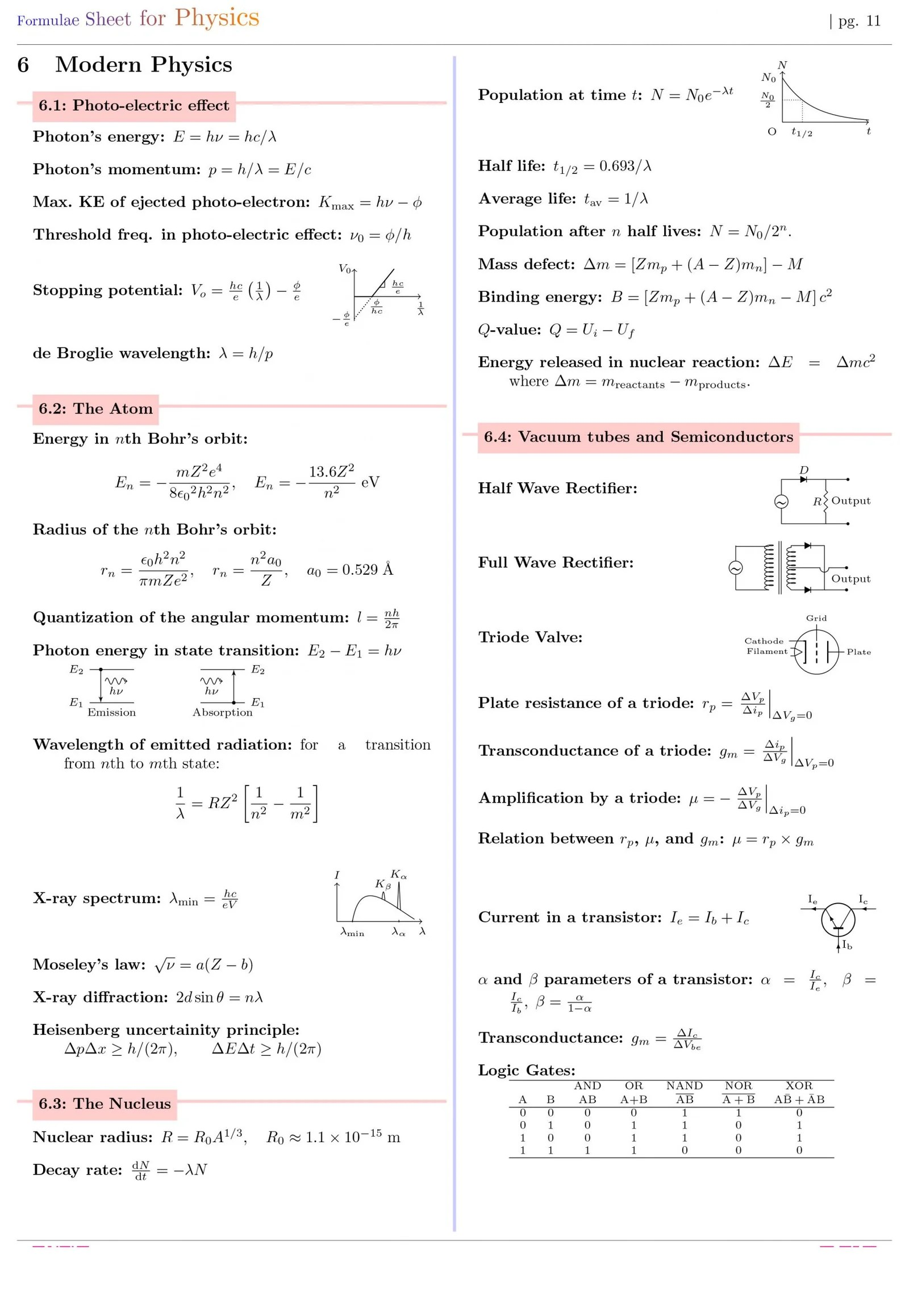# Formulae Sheet for Physics

0.1: Physical Constants
Speed of light c
Planck constant
Gravitation constant G
Boltzmann constant k
Molar gas constant R
Charge of electron e
Permeability of vacuum
Permitivity of vacuum
Coulomb constant
Mass of electron me
Mass of proton mp
Mass of neutron mn
Atomic mass unit u
Atomic mass unit u
Stefan-Boltzmann constant
Rydberg constant
Bohr magneton
Standard atmosphere
Wien displacement constant

1 MECHANICS

1.1: Vectors
Notation:
Magnitude:
Dot product:
Cross product:

1.2: Kinematics
Average and Instantaneous Vel. and Accel.:
Motion in a straight line with constant a:
Motion in a straight line with constant a:
Projectile Motion:

1.3: Newton’s Laws and Friction
Linear momentum:
Newton’s First law:
Newton’s second law:
Newton’s third law:
Frictional force:
Banking angle:
Centripetal force:
Pseudo force:
Minimum speed to complete vertical circle:
Conical pendulum:

1.4: Work, Power and Energy
Work:
Kinetic energy:
Potential energy:
Work done by conservative forces is path independent and depends only on initial and Final points:
Work-energy theorem:
Mechanical energy:
Power: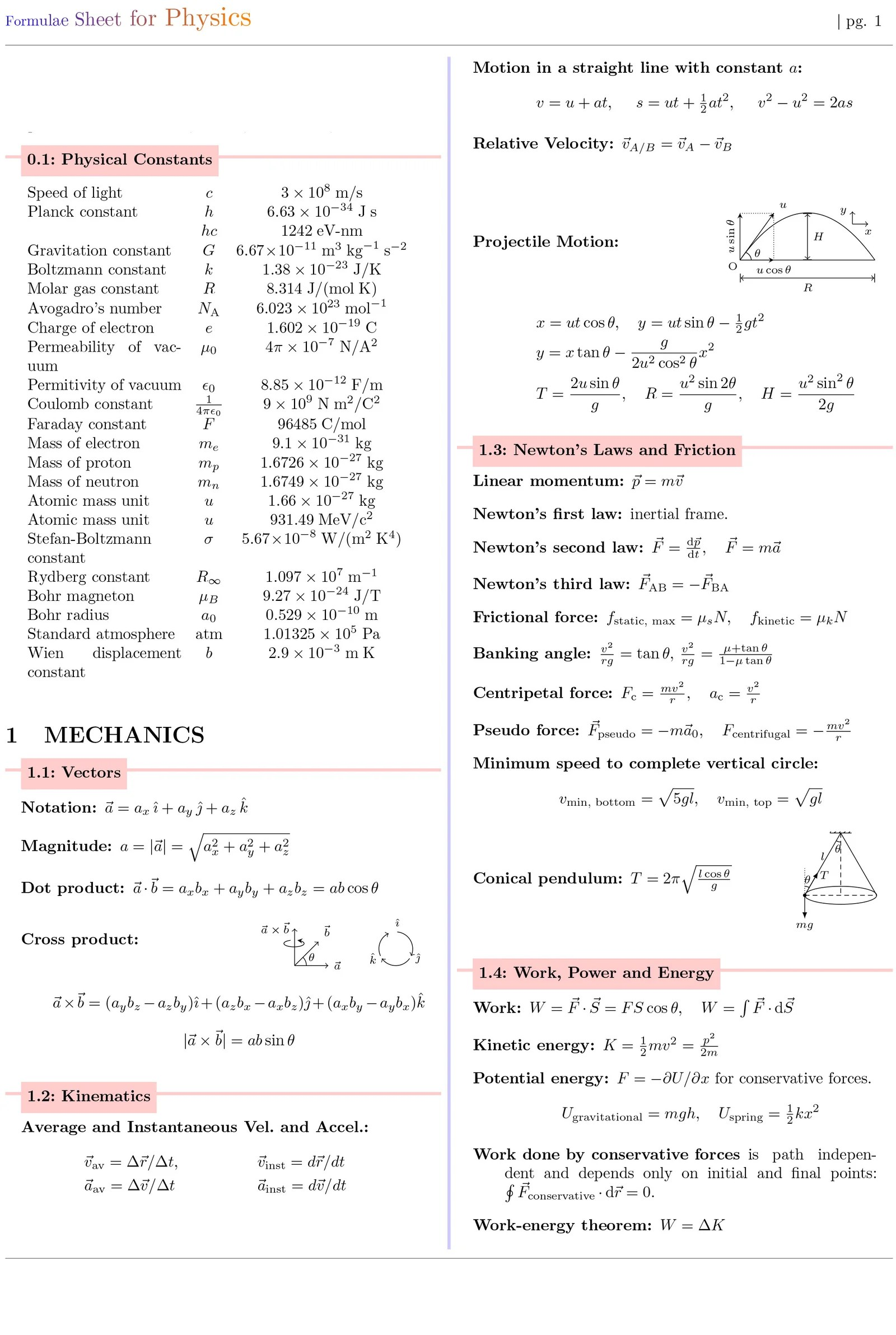1.5: Centre of Mass and Collision
Centre of mass:
CM of few useful configurations:
Motion of the CM:

1.6: Rigid Body Dynamics
Angular velocity:
Angular Accel.:
Rotation about an axis with constant:
Moment of Inertia:
Theorem of Parallel Axes:
Theorem of Perp. Axes:
Angular Momentum:
Torque:
Conservation of ~ L:
Equilibrium condition:
Kinetic Energy:
Dynamics:

1.7: Gravitation
Gravitational force:
Potential energy:
Gravitational acceleration:
Variation of g with depth:
Variation of g with height:
Effect of non-spherical earth shape on g:
Effect of earth rotation on apparent weight:
Orbital velocity of satellite:
Escape velocity:
Kepler’s laws: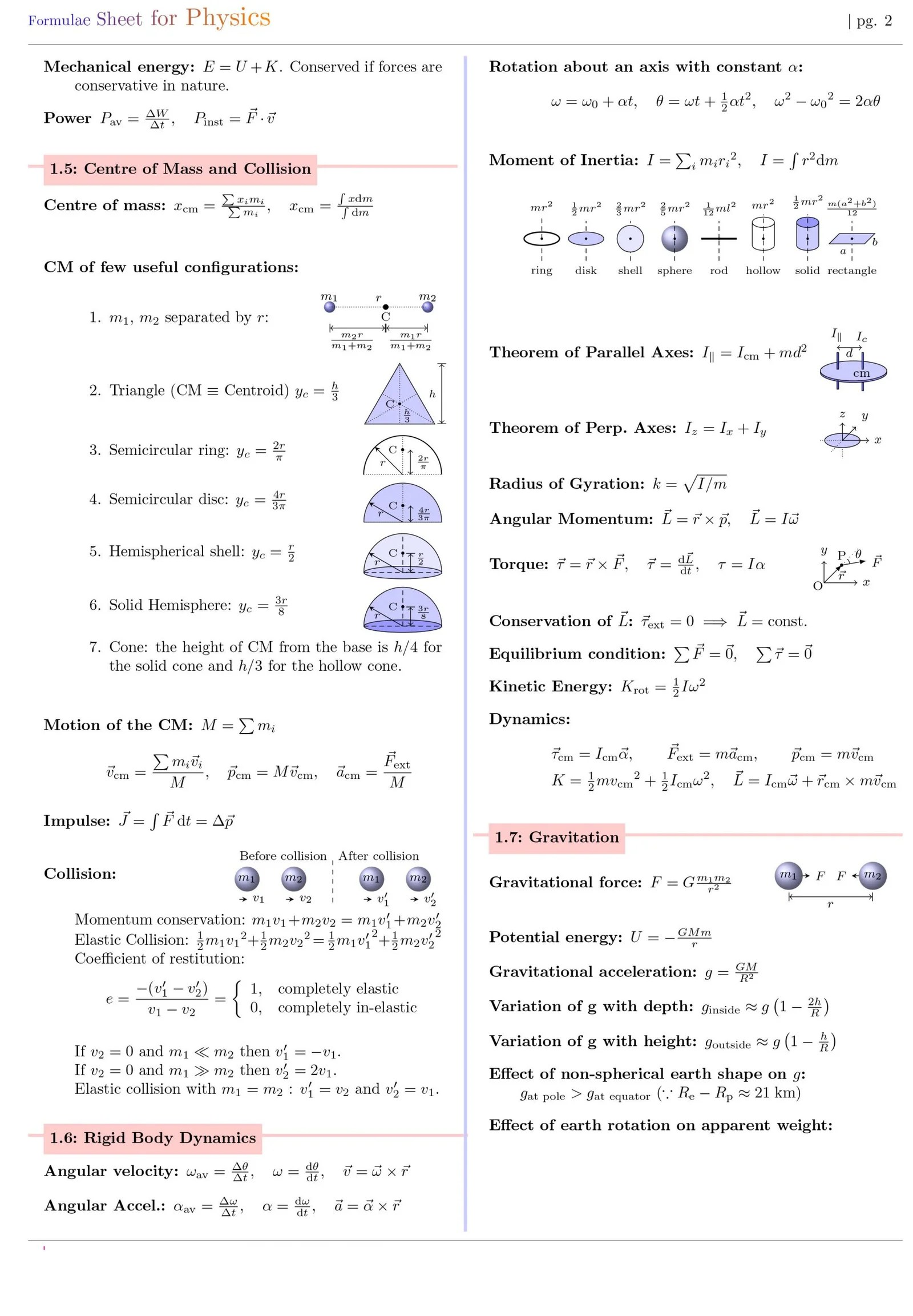1.8: Simple Harmonic Motion
Hooke’s law: F
Acceleration:
Time period:
Velocity: v
Potential energy:
Kinetic energy K
Total energy:
Simple pendulum: T
Physical Pendulum:
Torsional Pendulum
Springs in series:
Springs in parallel:
Superposition of two SHM’s:

1.9: Properties of Matter
Modulus of rigidity:
Compressibility:
Poisson’s ratio:
longitudinal strain
Elastic energy:
Surface tension:
Surface energy:
Excess pressure in bubble:
Capillary rise: h
Hydrostatic pressure:
Buoyant force:
Equation of continuity:
Bernoulli’s equation:
Torricelli’s theorem:
Viscous force:
Stoke’s law: F
Poiseuilli’s equation:
Terminal velocity: